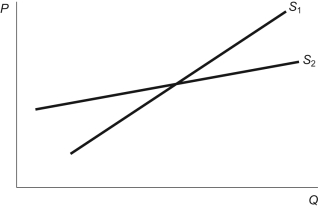# [Solved] Figure: Price Elasticity of Supply Refer to the Figure

Question 130
Multiple Choice

## Figure: Price Elasticity of SupplyRefer to the figure. Compared to S1, S2 is more:

A) elastic since quantity supplied increases more with an increase in price.
B) elastic since quantity supplied increases less with an increase in price.
C) inelastic since quantity supplied increases more with an increase in price.
D) inelastic since quantity supplied increases less with an increase in price.

10+ million students use Quizplus to study and prepare for their homework, quizzes and exams through 20m+ questions in 300k quizzes.

Explore our library and get Economics Homework Help with various study sets and a huge amount of quizzes and questions

3.5K

Study sets

66.5K

Quizzes

5.9M

Questions

Upload material to get free accessInvite a friend and get free accessSubscribe and get an instant access